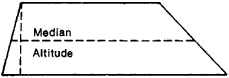# trapezoid

(redirected from trapezoid bone)
Also found in: Dictionary, Thesaurus, Medical, Wikipedia.
Related to trapezoid bone: scaphoid bone, capitate bone, trapezium bone

## trapezoid,

closed plane figure bounded by four line segments, or sides, two of which are parallel and two of which are nonparallel. The parallel sides of a trapezoid are called bases and the nonparallel sides legs; in an isosceles trapezoid the legs are of equal length. The median of a trapezoid is the line segment connecting the midpoints of the legs; it is parallel to the bases and equal to half the sum of their lengths. The altitude of a trapezoid is the perpendicular distance between the bases. The area of a trapezoid is equal to half the product of the altitude and the sum of the bases, i.e., to the product of the altitude and the median.

## Trapezoid

A four-sided figure with unequal sides; a parallel trapezoid has two unequal parallel sides and two equal nonparallel sides; a symmetrical trapezoid has two pairs of adjacent equal sides.
The following article is from The Great Soviet Encyclopedia (1979). It might be outdated or ideologically biased.

## Trapezoid

a convex quadrilateral having two parallel and two nonparallel sides (see Figure 1). The parallel sides are called the bases of the trapezoid, and the nonparallel sides are sometimes referred to as the trapezoid’s legs. The line segment joining the midpoints of the nonparallel sides is called the median, or midline. The median is parallel to the bases, and its length is equal to half the sum of their lengths. The area of a trapezoid is equal to the product of the trapezoid’s altitude and the length of its median. The area is also equal to half the product of the lengths of the diagonals and the sine of the angle between them. In an isosceles trapezoid, the nonparallel sides are of equal length.Figure 1

## trapezoid

[′trap·ə‚zȯid]
(mathematics)
A quadrilateral having two parallel sides.
McGraw-Hill Dictionary of Scientific & Technical Terms, 6E, Copyright © 2003 by The McGraw-Hill Companies, Inc.

## trapezoid

1. a quadrilateral having neither pair of sides parallel
2. US and Canadian a quadrilateral having two parallel sides of unequal length
3. a small bone of the wrist near the base of the index finger
Collins Discovery Encyclopedia, 1st edition © HarperCollins Publishers 2005
Site: Follow: Share:
Open / Close